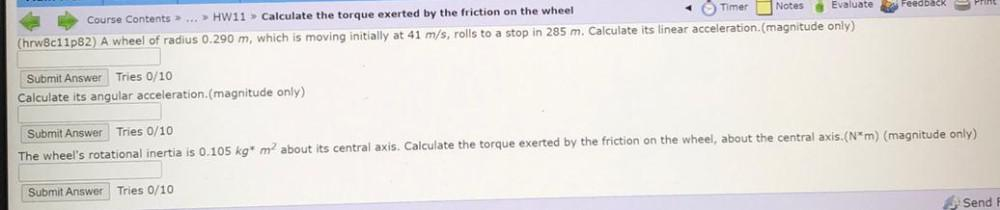Question:

# Course Contents> ... >> HW11 > Calculate the torque exerted by the friction on the wheel Timer Notes Evaluate Feedback Print (hrCourse Contents> ... >> HW11 > Calculate the torque exerted by the friction on the wheel Timer Notes Evaluate Feedback Print (hrw8c11p82) A wheel of radius 0.290 m, which is moving initially at 41 m/s, rolls to a stop in 285 m. Calculate its linear acceleration(magnitude only) Submit Answer Tries 0/10 Calculate its angular acceleration.(magnitude only) Submit Answer Tries 0/10 The wheel's rotational inertia is 0.105 kg* m about its central axis. Calculate the torque exerted by the friction on the wheel, about the central axis.(N*m) (magnitude only) Submit Answer Tries 0/10 Send